﻿ 一种不对称半桥正反激DC/DC变换器

# 一种不对称半桥正反激DC/DC变换器An Asymmetric Half Bridge Forward-Flyback Hybrid DC/DC Converter

Abstract: Based on the existed active clamp forward-flyback hybrid DC/DC, a new asymmetric half bridge forward-flyback hybrid DC/DC converter is proposed in the paper. The asymmetrical half bridge structure is applied to active clamp forward-flyback converter, which inherits the characteristics of the active clamp forward-flyback converter with high efficiency and high power density, and the voltage stress of the switch is greatly reduced. In this paper, the operating principle of the converter is discussed in detail; the key time sequence is given; and realization conditions of soft switching and basic quantitative relationship in steady state are analyzed emphatically. Based on the theoretical analysis, saber is used to do simulation analysis and make an 80 W experimental prototype to validate the validity of theoretical analysis.

1. 引言

2. 变换器工作原理

2.1. 变换器结构

2.2. 变换器工作原理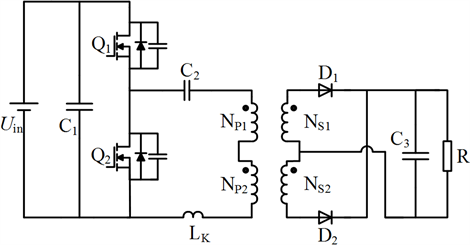Figure 1. AHB forward-flyback hybrid converter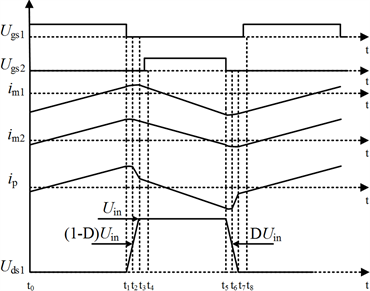(a) 等效线路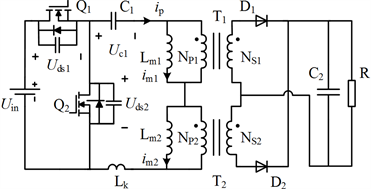(b) 工作波形

Figure 2. Equivalent circuit and key waveforms of the converter

1) 模态1 [t0-t1]：Q1处于开通状态，变压器原边电流线性上升。正激变压器T1向负载传输能量，D1导通，变压器T1原边电压被负载侧电压嵌位；反激变压器原边绕组做电感，D2关断，原边电流ip与励磁电流im2相等且线性上升，反激变压器储存能量。

2) 模态2 [t1-t2]：Q1关断，原边电流ip给CQ2放电，同时对CQ1充电，Uds1上升，Uds2持续下降。D1导通，D2保持关断。当Uds2下降到Uc1时，Uds1上升到Uin-Uc1，D2开始导通，D1和D2开始换流。寄生电容CQ1的存在可实现Q1的零电压关断。

3) 模态3 [t2-t3]：D1和D2都导通，两变压器原边电压都被负载侧电压嵌位。变压器漏感Lk与CQ1和CQ2谐振，Lk继续给谐振CQ2放电，给谐振CQ1充电。当Uds2下降到0 V，Uds1上升到Uin并被嵌位，Q2的体二极管DQ2开始导通续流。D1关断，D2开始持续导通。

4) 模态4 [t3-t4]：DQ2在此阶段持续导通，此时开通Q2可实现开关管零电压开通。

5) 模态5 [t4-t5]：Q2处于开通状态，反激变压器T2向负载传输能量，T2原边电压被输出电压嵌位。正激变压器T1原边承受反压，其值为Uc2-n2Uo，T1在这个电压的作用下磁芯复位。原边电流ip与T1的励磁电流im1相等，并且在C1反压的作用下减小然后反向。

6) 模态6 [t5-t6]：Q2关断，原边电流ip给CQ1放电，同时对CQ2充电。Uds2上升，Uds1持续下降。D2导通，D1保持关断。Uds1下降到Uin-Uc1，Uds2上升到Uc1时，D1开始导通，D1和D2开始换流。寄生电容CQ2的存在可实现Q2的零电压关断。

7) 模态7 [t6-t7]：D1和D2都导通，两变压器原边电压再次被负载侧电压嵌位。漏感Lk与CQ1和CQ2谐振，漏感Lk继续给CQ1谐振放电，给CQ2谐振充电。当Uds1下降到0，Uds2上升到Uin并被嵌位，Q1的体二极管DQ1开始导通续流。D2关断，D1开始持续导通。

8) 模态8 [t7-t8]：DQ1在此阶段持续导通，此时开通Q1可实现开关管零电压开通。

3. 稳态特性分析

3.1. 基本数量关系

1) 隔直电容电压

$\left({U}_{\text{in}}-{U}_{\text{cl}}\right)D{T}_{S}={U}_{\text{cl}}\left(1-D\right){T}_{S}$ (1)

${U}_{\text{cl}}=D{T}_{\text{in}}$ (2)

2) 输入输出关系

$\left({U}_{\text{in}}-{U}_{\text{cl}}-{n}_{1}{U}_{o}\right)D{T}_{S}={n}_{2}{U}_{o}\left(1-D\right){T}_{S}$ (3)

${U}_{o}=\frac{D\left(1-D\right)}{\left[{n}_{1}D+{n}_{2}\left(1-D\right)\right]}{U}_{\text{in}}$ (4)

${U}_{o}=\frac{D\left(1-D\right)}{n}{U}_{\text{in}}$ (5)

3) 器件应力分析

${U}_{\text{ds1}}={U}_{\text{ds2}}={U}_{\text{in}}$ (6)

${U}_{\text{ds1}}={U}_{\text{ds2}}=\frac{{U}_{\text{in}}}{1-D}$ (7)

${U}_{\text{d2}}=\frac{1-D}{n}{U}_{\text{in}}=\frac{{U}_{o}}{D}$ (8)

${U}_{\text{d1}}=\frac{D}{n}{U}_{\text{in}}=\frac{{U}_{o}}{1-D}$ (9)

3.2. 软开关条件

${i}_{p\mathrm{max}}=\frac{\left(1-D\right){U}_{\text{in}}-n{U}_{o}}{2{L}_{m2}}D{T}_{S}$ (10)

4. 仿真分析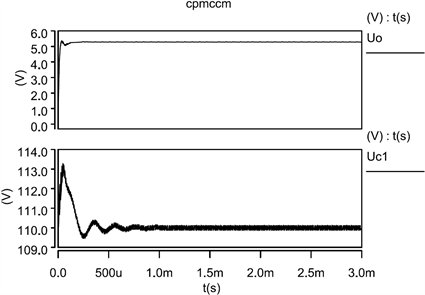Figure 3. Input-output relation and isolated capacitor voltage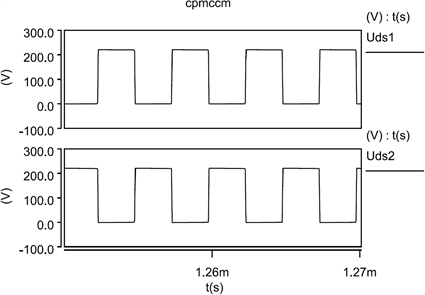Figure 4. Voltage stress of switch tube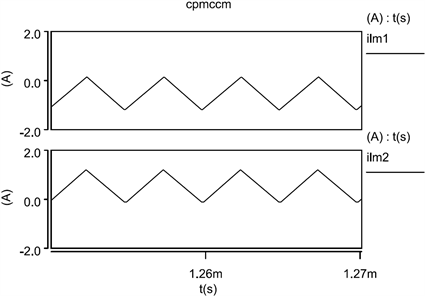Figure 5. The current of transformer excitation inductance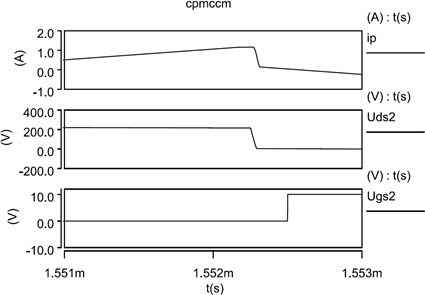Figure 6. Soft switching waveform of switch tube Q2

5. 实验验证

6. 结束语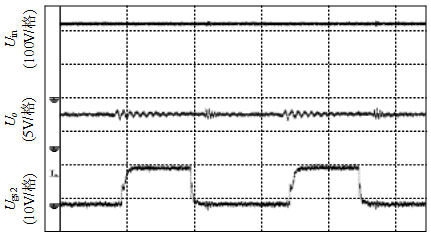Figure 7. Input and output waveforms of the converterFigure 8. Soft switching waveforms of the converter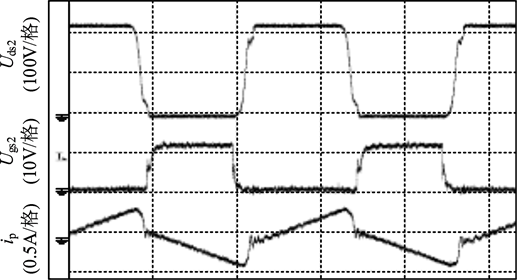Figure 9. Waveforms of primary side current ip and soft switching waveforms of Q2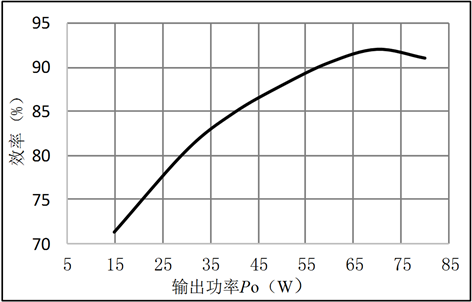Figure 10. Efficiency chart

 张方华, 严仰光. 一族正反激组合式双向DC-DC变换器[J]. 中国电机工程学报, 2004, 24(5): 157-162.

 张方华. 双向DC-DC变换器的研究[D]: [博士学位论文]. 南京: 南京航空航天大学, 2005.

 魏应冬, 吴建德, 顾亦磊. 一种新型双管正–反激直流变换器[J]. 中国电机工程学报, 2005, 25(20): 50-55.

 许晓慧, 苏义荣, 王双虎, 席旸旸, 姚虹春, 窦友婷. 基于电动汽车装置DC/DC变换器的研究[J]. 电测与仪表, 2016, 53(24): 110-114.

 Zhang, F.H., Xiao, L. and Yan, Y.G. (2004) Bi-Directional Forward-Flyback DC-DC Converter. 2004 IEEE 35th Annual Power Elec-tronics Specialists Conference, Aachen, 20-25 June 2004, 4058-4061.
https://doi.org/10.1109/PESC.2004.1355194

 胡红林, 李春华, 邵波. 移相全桥零电压PWM软开关电路的研究[J]. 电力电子技术, 2009, 43(1): 12-14.

 杜少武, 蒋劲松. 零电压零电流不对称半桥串联混合式直流变换器[J]. 中国电机工程学报, 2006, 26(24): 60-65.

 陈章勇, 许建平, 王金平, 张斐. 一种新型非调节隔离DC-DC变换器[J]. 电工技术学报, 2014, 29(12): 58-65+72.

 廖国虎, 邱国跃, 袁旭峰. 电力电子变压器研究综述[J]. 电测与仪表, 2014, 51(16): 5-10+36.

 李伟东. 大功率全桥移相软开关电源的研究[D]: [硕士学位论文]. 成都: 西南交通大学, 2004.

 张方华, 严仰光. 变压器匝比不同的正反激组合式双向DC-DC变换器[J]. 中国电机工程学报, 2005, 25(14): 57-61.

Top